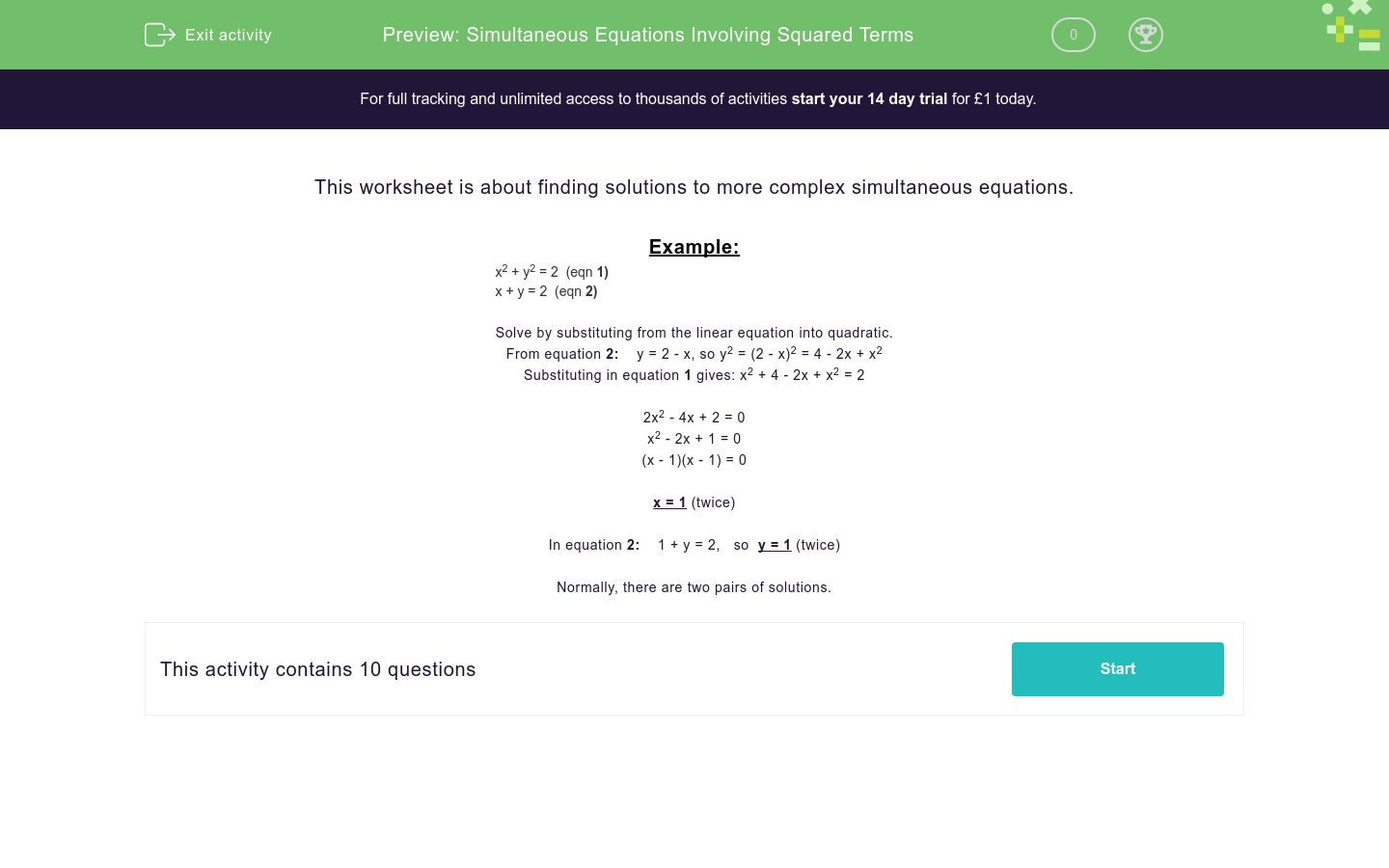# Simultaneous Equations Involving Squared Terms

In this worksheet, students solve simultaneous equations involving quadratic equationsKey stage:  KS 4

Curriculum topic:  Algebra

Curriculum subtopic:  Solve Simultaneous Equations in 2 Variables

Difficulty level:### QUESTION 1 of 10

This worksheet is about finding solutions to more complex simultaneous equations.

Example:

 x2 + y2 = 2  (eqn 1) x + y = 2  (eqn 2) Solve by substituting from the linear equation into quadratic. From equation 2:    y = 2 - x, so y2 = (2 - x)2 = 4 - 2x + x2 Substituting in equation 1 gives: x2 + 4 - 2x + x2 = 2 2x2 - 4x + 2 = 0 x2 - 2x + 1 = 0 (x - 1)(x - 1) = 0 x = 1 (twice) In equation 2:    1 + y = 2,   so  y = 1 (twice)   Normally, there are two pairs of solutions.

Solve the following simultaneous equations:

x2 + y2 = 8
x + y = 4

x = _______
y = _______

 x y 1 -1 2 -2

Solve the following simultaneous equations:

2x2 + 2y2 = 9
x + y = 3

x = _______
y = _______

 x y 1.5 -1 2.5 -2

Select one solution to these simultaneous equations:

 x = 3 y

y = 2x −5

x = _______
y = _______

 x y 1 2 3 4

Select one solution to this pair of simultaneous equations:

x2  = 3y
x + 6 = y

x = _______
y = _______

 x y 3 -1 2 -3

Solve the following simultaneous equations:

25 - 2x2  = 2y2
x  = 5 - y

x = _______
y = _______

 x y 3.5 -1 2.5 -3

Select one solution to this pair of simultaneous equations:

xy  = 5
2x - y = 9

x = _______
y = _______

 x y 1 3 5 7

Solve the following simultaneous equations:

18 - y2 = x2
y = 6 - x

x = _______
y = _______

 x y 1 2 3 4

Select one solution to this pair of simultaneous equations:

18 - 3y2 = -x
y = 6 - x

x = _______
y = _______

 x y 1 2 3 4

Select one solution to this pair of simultaneous equations:

25 - 2x2 = y- 9
x = 7 - y

x = _______
y = _______

 x y 1.5 2.5 3 4

Select one solution to this pair of simultaneous equations:

 x = 3 y

y = 3x − 8

x = _______
y = _______

 x y 1 2 3 4
• Question 1

Solve the following simultaneous equations:

x2 + y2 = 8
x + y = 4

x = _______
y = _______

 x y 1 -1 2 -2
• Question 2

Solve the following simultaneous equations:

2x2 + 2y2 = 9
x + y = 3

x = _______
y = _______

 x y 1.5 -1 2.5 -2
• Question 3

Select one solution to these simultaneous equations:

 x = 3 y

y = 2x −5

x = _______
y = _______

 x y 1 2 3 4
• Question 4

Select one solution to this pair of simultaneous equations:

x2  = 3y
x + 6 = y

x = _______
y = _______

 x y 3 -1 2 -3
• Question 5

Solve the following simultaneous equations:

25 - 2x2  = 2y2
x  = 5 - y

x = _______
y = _______

 x y 3.5 -1 2.5 -3
• Question 6

Select one solution to this pair of simultaneous equations:

xy  = 5
2x - y = 9

x = _______
y = _______

 x y 1 3 5 7
• Question 7

Solve the following simultaneous equations:

18 - y2 = x2
y = 6 - x

x = _______
y = _______

 x y 1 2 3 4
• Question 8

Select one solution to this pair of simultaneous equations:

18 - 3y2 = -x
y = 6 - x

x = _______
y = _______

 x y 1 2 3 4
• Question 9

Select one solution to this pair of simultaneous equations:

25 - 2x2 = y- 9
x = 7 - y

x = _______
y = _______

 x y 1.5 2.5 3 4
• Question 10

Select one solution to this pair of simultaneous equations:

 x = 3 y

y = 3x − 8

x = _______
y = _______